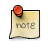Navigation C API Pages Python bindings Applications

## Gamma Correction

### What is gamma and what is it doing in my computer?

First of all gamma is a function, or better there is a gamma function and it’s inverse function. Both gamma function and it’s inverse are defined on interval [0,1] and are defined as out = in(gamma) and it’s inverse as out = in(1/gamma).

The purpose of this function is to compensate nonlinearity of human eye perception. The human eye is more sensitive to dark tones than the light ones so without gamma correction storage and manipulation with image data would either be less efficient in space (in case you decided to use more bits and encode the image linearly) or quantization in darker tones would be more visible resulting in "pixelated" images (aliasing).

So there is a gamma, the Internet seems to suggest that usual values for gamma are 2.5 for old CRT monitors and about 2.2 for LCD ones, ideally you should have color profile for your device (you need special hardware to measure it). So if you are trying to draw linear gradient on the screen you need to generate sequence of numbers accordingly to gamma function (the 50% intensity is around 186 for gamma = 2.2 and 8bit grayscale pixel).

Moreover image formats tend to save data in nonlinear fashion (some formats include gamma value used to for the image) so before you apply filter that manipulates with pixel values, you need to convert it to linear space (adding some more bits to compensate for rounding errors).

Also it’s important to take gamma, into an account, when drawing anti aliased shapes, you can’t get right results otherwise.The gamma support in GFXprim is quite new and at a time of writing this docs, only one filter does support gamma correction. (The anti-aliased drawing and text still use legacy gamma support.)

### Implementation

The gp_gamma structure defines per pixmap, per channel, gamma tables.

The tables for particular gamma are reference counted. There is only one table for particular gamma value and bit depth in memory at a time.

Also the table output, for linear values, has two more bits than original in order not to loose precision.

The pointers to gamma tables are storied in gp_gamma structure and are organized in the same order as channels. First N tables for each channel and gamma value gamma, then N tables for inverse 1/gamma function.

So when we have RGB888 pixel and gamma 2.2 there are two tables in the memory, one for gamma 2.2 input 8bit output 10bit and it’s inverse input 10bit output 8bit. The gp_gamma contains six pointers. First three points to the gamma table for gamma 2.2 with 8bit input (256 array members) and the output format is 10bits so each array member is uint16_t. The other three are for inverse gamma function (gamma = 0.454545…) with 10bit input (1024 array members) and 8bit output so each member is uint8_t.

The whole interface is designed for speed, so that conversion to linear space or from linear space is just a matter of indexing arrays. Imagine you need to get gamma-corrected pixel value. First you take individual pixel channels then use the gp_gamma structure as follows:

```
/* To convert channel gamma value to linear value: */
gamma->tables[chan_number].u16[chan_val]

/* ... or when result has no more than 8bits ... */

gamma->tables[chan_number].u8[chan_val]

/* The inverse transformation is done as: */

gamma->tables[chan_count + chan_number].u8[chan_val]

/* ... or when original pixel channel had more than 8bits ... */

gamma->tables[chan_count + chan_number].u16[chan_val]

/*
* When doing more than one conversion it's better to save pointers to
* individual table (example for RGB888):
*/

uint16_t *R_2_LIN = gamma->tables.u16;
/* ... */
uint8_t *R_2_GAMMA = gamma->tables.u8;
/* ... */
```

### Gamma low level API

```#include <core/gp_gamma.h>
/* or */
#include <gfxprim.h>

gp_gamma *gp_gamma_acquire(gp_pixel_type pixel_type, float gamma);```

Returns pointer to gamma table for particular pixel_type and gamma value.

The same gamma is used for all channels.

May fail and return NULL if malloc() has failed.

```#include <core/gp_gamma.h>
/* or */
#include <gfxprim.h>

gp_gamma *gp_gamma_copy(gp_gamma *gamma);```

Copies a Gamma table (actually only increases ref_count).

Can’t fail.

```#include <core/gp_gamma.h>
/* or */
#include <gfxprim.h>

void gp_gamma_release(gp_gamma *self);```

Releases Gama table (if ref_count has fallen to zero, frees memory).